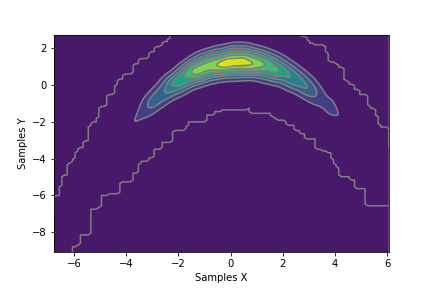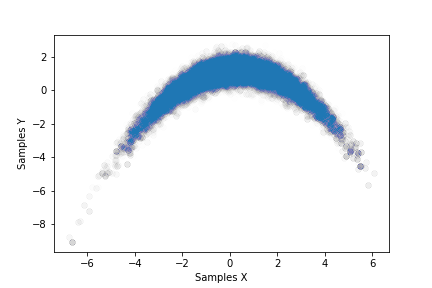# Analytic Banana¶

## Overview¶

This benchmark consists of an analytically defined PDF $$\pi : \mathbb{R}^2 \rightarrow \mathbb{R}$$ resembling the shape of a banana. It is based on a transformed normal distribution. The variance may be adjusted.## Run¶

docker run -it -p 4243:4243 linusseelinger/benchmark-analytic-banana


## Properties¶

Model

Description

posterior

Posterior density

### posterior¶

Mapping

Dimensions

Description

input



2D coordinates $$x \in \mathbb{R}^2$$

output



Log PDF $$\pi$$ evaluated at $$x$$

Feature

Supported

Evaluate

True

False

ApplyJacobian

False

ApplyHessian

False

Config

Type

Default

Description

a

double

2.0

Transformation parameter

b

double

0.2

Transformation parameter

scale

double

1.0

Scaling factor applied to the underlying normal distribution’s variance

Mount directory

Purpose

None

## Source code¶

Model sources here.

## Description¶

We begin with a normally distributed random variable $$Z \sim \mathcal{N}(\begin{pmatrix} 0 \\ 4 \end{pmatrix}, scale \begin{pmatrix} 1.0 & 0.5\\ 0.5 & 1.0 \end{pmatrix})$$, and denote its PDF by $$f_Z$$.

In order to reshape the normal distribution, define a transformation $$T : \mathbb{R}^2 \rightarrow \mathbb{R}^2$$

$\begin{split} T(x) := \begin{pmatrix} x_1 / a \\ a x_2 + a b (x_1^2 + a^2) \end{pmatrix}. \end{split}$

Finally, the benchmark log PDF is defined as

$log(\pi(x)) := log(f_Z(T(x))).$

This distribution is inspired by Chi Feng’s excellent online mcmc-demo.